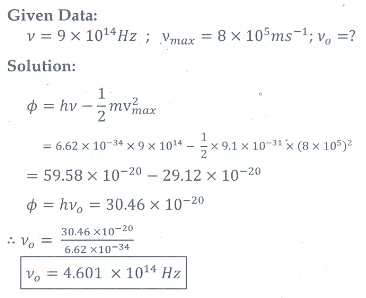Home | | Physics 12th Std | Exercise Numerical problems

# Exercise Numerical problems

Physics : Dual Nature of Radiation and Matter : Book Back, Exercise, Example Numerical Question with Answers, Solution: Exercise Numerical problems

IV Numerical problems

1. How many photons per second emanate from a 50 mW laser of 640 nm?

[Ans:1.61├Ś1017 sŌłÆ1 ]2. Calculate the maximum kinetic energy and maximum velocity of the photoelectrons emitted when the stopping potential is 81for the photoelectric emission experiment.

[[Ans: 1.3├Ś10ŌĆō17J; 5.3├Ś106 msŌĆō1]]3. Calculate the energies of the photons associated with the following radiation: (i) violet light of 413 nm (ii) X-rays of 0.1 nm (iii) radio waves of 10 m.

[Ans: 3eV ; 12424eV ;1.24├Ś10ŌłÆ7 eV ]4. A 150 lamp emits light of mean wavelength of 5500 ├ģ . If the efficiency is 12%, find out the number of photons emitted by the lamp in one second.

[Ans: 4.98├Ś1019 ]5. How many photons of frequency 1014 Hz will make up 19.86 of energy?

[Ans: 3┬┤1020 ]6. What should be the velocity of the electron so that its momentum equals that of 4000 ├ģ wavelength photon.

[Ans: 1818ms-1 ]7. When a light of frequency 9┬┤1014 Hz is incidentona metal surface, photoelectrons are emitted with a maximum speed of 8├Ś105 msŌłÆ1 . Determine the threshold frequency of the surface.

[Ans: 4.61┬┤1014 Hz ]8. When a 6000 ├ģ light falls on the cathode of a photo cell and produced photoemission. If a stopping potential of 0.8 is required to stop emission of electron, then determine the (i) frequency of the light (ii) energy of the incident photon (iii) work function of the cathode material (iv) threshold frequency and (v) net energy of the electron after it leaves the surface.

[Ans: 5├Ś1014 Hz; 2.07 eV; 1.27 eV; 3.07├Ś1014 Hz; 0.8 eV ]9. A 3310 ├ģ photon liberates an electron from a material with energy 3├Ś10ŌłÆ19 while another 5000 ├ģ photon ejects an electron with energy 0.972├Ś10ŌłÆ19 from the same material. Determine the value of PlanckŌĆÖs constant and the threshold wavelength of the material.

[Ans: 6.62├Ś10ŌłÆ34 Js; 6620├Ś10ŌłÆ10 ]10. At the given point of time, the earth receives energy from sun at 4 cal cm-2 min-1. Determine the number of photons received on the surface of the Earth per cm2 per minute. (Given : Mean wavelength of sun light = 5500 ├ģ )

[Ans: 4.65┬┤1019 ]11. UV light of wavelength 1800 ├ģ is incident on a lithium surface whose threshold wavelength 4965 ├ģ . Determine the maximum energy of the electron emitted.

[Ans: 4.40 eV]12. Calculate the de Broglie wavelength of a proton whose kinetic energy is equal to 81.9 ├Ś 10ŌĆō15 J. (Given: mass of proton is 1836 times that of electron).

[Ans: 4├Ś10ŌłÆ14 ]13. A deuteron and an alpha particle are accelerated with the same potential. Which one of the two has i) greater value of de Broglie wavelength associated with it and ii) less kinetic energy? Explain.

[Ans: ╬╗d = 2╬╗╬▒ and Kd KdKd/2 ]14. An electron is accelerated through a potential difference of 81V. What is the de Broglie wavelength associated with it? To which part of electromagnetic spectrum does this wavelength correspond?

[Ans: ╬╗ =1.36 ├ģ and x-rays]15. The ratio between the de Broglie wavelengths associated with protons, accelerated through a potential of 512 and that of alpha particles accelerated through a potential of volts is found to be one. Find the value of X.

[Ans: 64 V]Tags : with Answers, Solution | Dual Nature of Radiation and Matter | Physics , 12th Physics : UNIT 8 : Dual Nature of Radiation and Matter
Study Material, Lecturing Notes, Assignment, Reference, Wiki description explanation, brief detail
12th Physics : UNIT 8 : Dual Nature of Radiation and Matter : Exercise Numerical problems | with Answers, Solution | Dual Nature of Radiation and Matter | Physics